Site map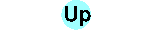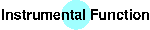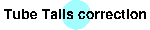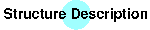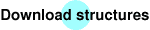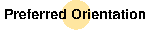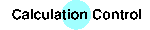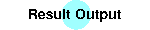A phenomenon occuring in nearly every sample is the preferred orientation (PO). Because we cannot avoid this we have to provide a model for its description. BGMN uses the spherical harmonics model up to the 10th order which allows the refinement of multiple and strong PO.

If one phase exhibits PO, a simple statement is necessary:

GEWICHT=SPHARx

x determines the order of the spherical harmonics model and can be 0, 2, 4, 6, 8 or 10. Except the triclinic lattice there are constraints between the parameters of the model. BGMN itself determines these constraints automatically.

Lets have a look at the gypsum structure file, lines 5 and 6:

PHASE=Gypsum SpacegroupNo=15 HermannMauguin=I12/a1 //
PARAM=A=0.6522_0.645^0.658 PARAM=B=1.5202_1.51^1.53 //
PARAM=C=0.5679_0.56^0.575 PARAM=BETA=118.4_118^119 //
RP=3 PARAM=B1=0_0^0.008 //
GEWICHT=SPHAR6 GOAL:gypsum=GEWICHT
E=CA+2 Wyckoff=e TDS=0.0088 y=0.4213
E=S    Wyckoff=e TDS=0.0066 y=0.9216
E=O-2  Wyckoff=f TDS=0.0149 x=0.5505 y=0.1319 z=0.9632
E=O-2  Wyckoff=f TDS=0.0141 x=0.6671 y=0.0223 z=0.7582
E=O-2  Wyckoff=f TDS=0.0219 x=0.4588 y=0.1821 z=0.3796
There is the GEWICHT=SPHAR6 statement in line 5 which determines the use of a 6th order spherical harmonics model.

If the phase content is too low, the order of the model will be reduced down to SPHAR4, SPHAR2 or even SPHAR0 (isotropy), if necessary. Thats why you can use the same structure file for all analytical problems even if nearly nothing of the phase is contained in the sample.